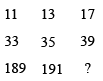Question 6

# Study the given pattern carefully and select the number that can replace the question mark (?) in it.Solution

The logic here is

Along first row

11 + 2 = 13

13 + 4 = 17

Along second row

33 + 2 = 35

35 + 4 = 39

Along the rows, second number is the sum of first number and 2, third number is the sum of second number and 4.

Similarly,

Along the third row

189 + 2 = 191

191 + 4 = 195

$$\therefore\$$The required number = 195

Hence, the correct answer is Option D

• Free SSC Study Material - 18000 Questions
• 230+ SSC previous papers with solutions PDF